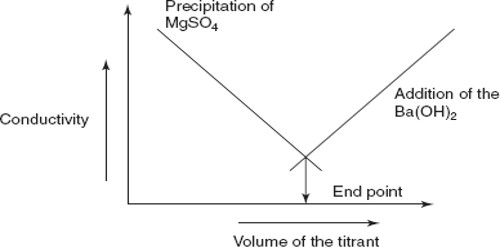Chemistry

# Conductance Titration in Precipitation ReactionsConductance titration in precipitation reactions:

In a precipitation reaction there is a net decrease in the number of ions in the solution due to removal of the ions from both the reacting substances. For example, when a solution of MgSO4 is added to a solution of Ba(OH)2 the Ba2+, ions and SO42+ are removed as BaSO4 and Mg2+ ions and OH are removed as Mg(OH)2 according to the equation:

Ba2+ (aq) + 2 OH (aq) + Mg2+ (aq) + SO42- (aq) = BaSO4 (s) + Mg(OH)2 (s)

Therefore, upon addition of a solution of MgSO4 to Ba(OH)2 solution there is a sharp fall in the conductance and this continues until the equivalence point is reached as shown in Figure.

However, after the equivalence point is exceeded, the conductance raises due to the contribution of Mg2+ and SO42- ions from the added MgSO4 solution. The method can be used for quantitative estimation of either Ba2+, Mg2+ or SO42-. In several other precipitation reactions the same method can be used and no indicators are needed.Fig: Precipitation titration by conductance measurements

Conductance titrations can be used for quantitative estimations of cations and anions in a variety of reactions. The principle, in all cases, is the change in the conductance of the reaction system due to addition or removal of some ions.